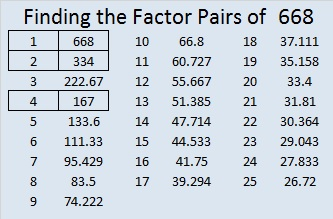# 668 and Level 3

668 is the sum of consecutive prime numbers 331 and 337.

68 is divisible by 4 so 668 and every other number ending in 68 is divisible by 4.

80 + 81 + 82 + 83 + 84 + 85 + 86 + 87 = 668Print the puzzles or type the solution on this excel file: 12 Factors 2015-11-02

—————————————————————————————————

• The first ten multiples of 668 are 668, 1336, 2004, 2672, 3340, 4008, 4676, 5344, 6012, and 6680.
• 668 is a composite number.
• Prime factorization: 668 = 2 x 2 x 167, which can be written 668 = (2^2) x 167
• The exponents in the prime factorization are 2 and 1. Adding one to each and multiplying we get (2 + 1)(1 + 1) = 3 x 2  = 6. Therefore 668 has exactly 6 factors.
• Factors of 668: 1, 2, 4, 167, 334, 668
• Factor pairs: 668 = 1 x 668, 2 x 334, or 4 x 167
• Taking the factor pair with the largest square number factor, we get √668 = (√4)(√167) = 2√167 ≈ 25.845696.—————————————————————————————————

A Logical Approach to solve a FIND THE FACTORS puzzle: Find the column or row with two clues and find their common factor. (None of the factors are greater than 12.)  Write the corresponding factors in the factor column (1st column) and factor row (top row).  Because this is a level three puzzle, you have now written a factor at the top of the factor column. Continue to work from the top of the factor column to the bottom, finding factors and filling in the factor column and the factor row one cell at a time as you go.